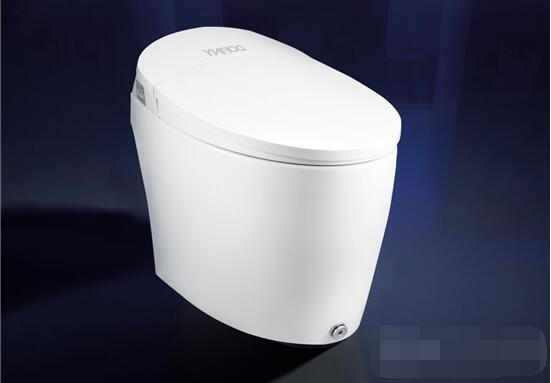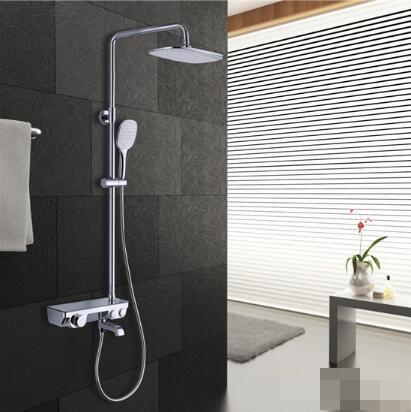|

# 智造改变生活 杜菲尼开启健康智能新时代

用“风起云涌”、“变幻莫测”来形容近两年的卫浴行业一点都不夸张。原材料上涨、经济结构调整、消费者结构升级、消费市场日新月异等等一系列问题席卷了整个卫浴行业，如何在这样的困境中突破重围杀出一条属于自己的“血路”，成为很多卫浴品牌的重中之重。扩大生产线、联合众筹、寻求资本、转型覆盖其他领域等等“大招”层出不穷。而这其中，却有一些企业不急不躁地站稳了脚跟，甚至找到了自己前行的方向。

对一个卫浴企业来说，最核心最关键的要素是什么？整体卫浴品牌杜菲尼认为，技术，是一个企业哪怕经过多少年也不能抛弃的核心关键，这既是一个企业不可改变赖以生存的基石，也是企业得以发展成长的保障。只有在技术领域把握优势，不断创新研发，才能以“不变”的姿态，来抵御市场上的任何变化。

随着“中国制造”加速向“中国智造”转变，如何牢牢把握住技术这个根本，寻找到适合自己的方向，杜菲尼在潜心专研后有了自己的答案。健康，作为被越来越多人关注的焦点，在卫浴空间的重要性正越来越凸显。杜菲尼认为，作为与人体0距离亲密接触的坐便器，哪怕是加上了“智能”的砝码，也应该将健康作为其最关注的问题。因此，杜菲尼加大对坐便器各材质、功能上的“健康”要素，打造了一系列新品，在完美诠释了其“健康卫浴专家”称号的同时，为人们带来了追求健康的新标准。

DB1807智能坐便器

医用级紫外线杀菌技术的率先运用

杜菲尼近期推出的新品智能坐便器DB1807，在目前智能坐便器喷头自洁技术的基础上更“净”一步，率先将医用级紫外线杀菌技术运用在了喷头上，在提高杀菌速度同时，使杀菌的范围扩大了。整个消毒的过程，既不受水质、水温的影响，又没有引进任何杂质和产生任何异味。该技术的突破，可以说完全满足了消费者对卫浴产品的奢想追求。DB1807智能坐便器

7大核心技术打造

DB1805N智能坐便器“笑傲群雄”

仅仅是在“净”上的更进一步尚且还不能称为一场技术盛宴。在杜菲尼同步推出的另一款新品智能坐便器DB1805N上，所展现出的技术升级和突破才真正令人叹为观止。

DB1805N采用的0-TOUCH零触技术，在完全解放了双手同时，让你不论是坐下还是起身都无需弯腰，只需脚尖轻轻一碰，即可完成自动翻盖和冲水两个动作，使你再也不用担心忘记冲水这种小事。DB1805N智能坐便器

除此之外，DB1805N还在7大核心技术上进行了更新和升级。其中，自动感应系统将自动冲洗、自动洁净、自动除臭、自动加热等一系列自动感应功能完全覆盖，让DB1805N达到了人工智能的产品范畴，贴心至极。瞬间加热技术的支持，让座圈的温度三挡可调，当座圈温度明显低于人体温度时，采用低温防灼伤技术的智能感应加热，从座圈上对你呵护备至。灯光感知技术在座圈上的运用，将环保节能的LED光源运用到座圈上，灯光柔和不刺眼，满足夜间如厕需要的同时还避免对眼睛造成刺激致使难以入睡。

另外，还有无水箱分水阀专利技术做到的空气隔离，真正做到了防虹吸和防逆流。再加上超强净化技术、app智能遥控、360°遥控技术等多项专利核心技术的共同支持，让杜菲尼仅凭DB1805N这一款产品便可在技术上“笑傲群雄”。

花洒技术再升级

恒温、增压、自洁三“花”齐放

健康专家杜菲尼显然并不满足于仅在智能马桶这一个品类上的专研。这从她们近期推出的几款花洒中就可窥见一斑。

以增压为主打的DLL32633花洒，对空气的混合让出水更加迅猛，在不刺痛皮肤的前提下， 还可达到节约相当于普通花洒40％的出水量；以自洁为主打的DLL32635花洒，运用重力机械的原理，使用时顶针上浮，关闭后顶针下落，达到了对顶喷的进行及时清洁的效果。以恒温为主打的DLL32903花洒，内置恒温阀芯和自带识别的按键，再也不用担心水温忽冷忽热的同时操作方便快捷。另外，此款花洒还拥有超大的置物平台，满足你对沐浴用品随时取用的需求。DLL32633增压花洒DLL32635自洁花洒DLL32903恒温花洒

杜菲尼以健康专家的身份，在卫浴这个领域以技术打造的这场新品盛宴，充分展示了这个整体卫浴专家在研发领域的强大和对新技术新产品的创新尝试，并开启了卫浴行业在健康追求上的更进一步。随着现今社会对健康的越发看中，消费者在产品的选择上也会在健康上更有倾向性，这从市场反馈回来的这些产品的销量和口碑上可见一斑。由此可见，杜菲尼抓住的不仅仅是技术这个“不变”的基石，更抓住了健康这个未来的主旋律，正在行业先锋的道路上稳步向前。

`声明：本文由入驻焦点开放平台的作者撰写，除焦点官方账号外，观点仅代表作者本人，不代表焦点立场错误信息举报电话： 400-099-0099，邮箱：jubao@vip.sohu.com，或点此进行意见反馈，或点此进行举报投诉。`A B C D E F G H J K L M N P Q R S T W X Y Z
A - B - C - D - E
• A
• 鞍山
• 安庆
• 安阳
• 安顺
• 安康
• 澳门
• B
• 北京
• 保定
• 包头
• 巴彦淖尔
• 本溪
• 蚌埠
• 亳州
• 滨州
• 北海
• 百色
• 巴中
• 毕节
• 保山
• 宝鸡
• 白银
• 巴州
• C
• 承德
• 沧州
• 长治
• 赤峰
• 朝阳
• 长春
• 常州
• 滁州
• 池州
• 长沙
• 常德
• 郴州
• 潮州
• 崇左
• 重庆
• 成都
• 楚雄
• 昌都
• 慈溪
• 常熟
• D
• 大同
• 大连
• 丹东
• 大庆
• 东营
• 德州
• 东莞
• 德阳
• 达州
• 大理
• 德宏
• 定西
• 儋州
• 东平
• E
• 鄂尔多斯
• 鄂州
• 恩施
F - G - H - I - J
• F
• 抚顺
• 阜新
• 阜阳
• 福州
• 抚州
• 佛山
• 防城港
• G
• 赣州
• 广州
• 桂林
• 贵港
• 广元
• 广安
• 贵阳
• 固原
• H
• 邯郸
• 衡水
• 呼和浩特
• 呼伦贝尔
• 葫芦岛
• 哈尔滨
• 黑河
• 淮安
• 杭州
• 湖州
• 合肥
• 淮南
• 淮北
• 黄山
• 菏泽
• 鹤壁
• 黄石
• 黄冈
• 衡阳
• 怀化
• 惠州
• 河源
• 贺州
• 河池
• 海口
• 红河
• 汉中
• 海东
• 怀来
• I
• J
• 晋中
• 锦州
• 吉林
• 鸡西
• 佳木斯
• 嘉兴
• 金华
• 景德镇
• 九江
• 吉安
• 济南
• 济宁
• 焦作
• 荆门
• 荆州
• 江门
• 揭阳
• 金昌
• 酒泉
• 嘉峪关
K - L - M - N - P
• K
• 开封
• 昆明
• 昆山
• L
• 廊坊
• 临汾
• 辽阳
• 连云港
• 丽水
• 六安
• 龙岩
• 莱芜
• 临沂
• 聊城
• 洛阳
• 漯河
• 娄底
• 柳州
• 来宾
• 泸州
• 乐山
• 六盘水
• 丽江
• 临沧
• 拉萨
• 林芝
• 兰州
• 陇南
• M
• 牡丹江
• 马鞍山
• 茂名
• 梅州
• 绵阳
• 眉山
• N
• 南京
• 南通
• 宁波
• 南平
• 宁德
• 南昌
• 南阳
• 南宁
• 内江
• 南充
• P
• 盘锦
• 莆田
• 平顶山
• 濮阳
• 攀枝花
• 普洱
• 平凉
Q - R - S - T - W
• Q
• 秦皇岛
• 齐齐哈尔
• 衢州
• 泉州
• 青岛
• 清远
• 钦州
• 黔南
• 曲靖
• 庆阳
• R
• 日照
• 日喀则
• S
• 石家庄
• 沈阳
• 双鸭山
• 绥化
• 上海
• 苏州
• 宿迁
• 绍兴
• 宿州
• 三明
• 上饶
• 三门峡
• 商丘
• 十堰
• 随州
• 邵阳
• 韶关
• 深圳
• 汕头
• 汕尾
• 三亚
• 三沙
• 遂宁
• 山南
• 商洛
• 石嘴山
• T
• 天津
• 唐山
• 太原
• 通辽
• 铁岭
• 泰州
• 台州
• 铜陵
• 泰安
• 铜仁
• 铜川
• 天水
• 天门
• W
• 乌海
• 乌兰察布
• 无锡
• 温州
• 芜湖
• 潍坊
• 威海
• 武汉
• 梧州
• 渭南
• 武威
• 吴忠
• 乌鲁木齐
X - Y - Z
• X
• 邢台
• 徐州
• 宣城
• 厦门
• 新乡
• 许昌
• 信阳
• 襄阳
• 孝感
• 咸宁
• 湘潭
• 湘西
• 西双版纳
• 西安
• 咸阳
• 西宁
• 仙桃
• 西昌
• Y
• 运城
• 营口
• 盐城
• 扬州
• 鹰潭
• 宜春
• 烟台
• 宜昌
• 岳阳
• 益阳
• 永州
• 阳江
• 云浮
• 玉林
• 宜宾
• 雅安
• 玉溪
• 延安
• 榆林
• 银川
• Z
• 张家口
• 镇江
• 舟山
• 漳州
• 淄博
• 枣庄
• 郑州
• 周口
• 驻马店
• 株洲
• 张家界
• 珠海
• 湛江
• 肇庆
• 中山
• 自贡
• 资阳
• 遵义
• 昭通
• 张掖
• 中卫

1室1厅1厨1卫1阳台

1
2
3
4
5

0
1
2

1

1

0
1
2
3报名成功，资料已提交审核A B C D E F G H J K L M N P Q R S T W X Y Z
A - B - C - D - E
• A
• 鞍山
• 安庆
• 安阳
• 安顺
• 安康
• 澳门
• B
• 北京
• 保定
• 包头
• 巴彦淖尔
• 本溪
• 蚌埠
• 亳州
• 滨州
• 北海
• 百色
• 巴中
• 毕节
• 保山
• 宝鸡
• 白银
• 巴州
• C
• 承德
• 沧州
• 长治
• 赤峰
• 朝阳
• 长春
• 常州
• 滁州
• 池州
• 长沙
• 常德
• 郴州
• 潮州
• 崇左
• 重庆
• 成都
• 楚雄
• 昌都
• 慈溪
• 常熟
• D
• 大同
• 大连
• 丹东
• 大庆
• 东营
• 德州
• 东莞
• 德阳
• 达州
• 大理
• 德宏
• 定西
• 儋州
• 东平
• E
• 鄂尔多斯
• 鄂州
• 恩施
F - G - H - I - J
• F
• 抚顺
• 阜新
• 阜阳
• 福州
• 抚州
• 佛山
• 防城港
• G
• 赣州
• 广州
• 桂林
• 贵港
• 广元
• 广安
• 贵阳
• 固原
• H
• 邯郸
• 衡水
• 呼和浩特
• 呼伦贝尔
• 葫芦岛
• 哈尔滨
• 黑河
• 淮安
• 杭州
• 湖州
• 合肥
• 淮南
• 淮北
• 黄山
• 菏泽
• 鹤壁
• 黄石
• 黄冈
• 衡阳
• 怀化
• 惠州
• 河源
• 贺州
• 河池
• 海口
• 红河
• 汉中
• 海东
• 怀来
• I
• J
• 晋中
• 锦州
• 吉林
• 鸡西
• 佳木斯
• 嘉兴
• 金华
• 景德镇
• 九江
• 吉安
• 济南
• 济宁
• 焦作
• 荆门
• 荆州
• 江门
• 揭阳
• 金昌
• 酒泉
• 嘉峪关
K - L - M - N - P
• K
• 开封
• 昆明
• 昆山
• L
• 廊坊
• 临汾
• 辽阳
• 连云港
• 丽水
• 六安
• 龙岩
• 莱芜
• 临沂
• 聊城
• 洛阳
• 漯河
• 娄底
• 柳州
• 来宾
• 泸州
• 乐山
• 六盘水
• 丽江
• 临沧
• 拉萨
• 林芝
• 兰州
• 陇南
• M
• 牡丹江
• 马鞍山
• 茂名
• 梅州
• 绵阳
• 眉山
• N
• 南京
• 南通
• 宁波
• 南平
• 宁德
• 南昌
• 南阳
• 南宁
• 内江
• 南充
• P
• 盘锦
• 莆田
• 平顶山
• 濮阳
• 攀枝花
• 普洱
• 平凉
Q - R - S - T - W
• Q
• 秦皇岛
• 齐齐哈尔
• 衢州
• 泉州
• 青岛
• 清远
• 钦州
• 黔南
• 曲靖
• 庆阳
• R
• 日照
• 日喀则
• S
• 石家庄
• 沈阳
• 双鸭山
• 绥化
• 上海
• 苏州
• 宿迁
• 绍兴
• 宿州
• 三明
• 上饶
• 三门峡
• 商丘
• 十堰
• 随州
• 邵阳
• 韶关
• 深圳
• 汕头
• 汕尾
• 三亚
• 三沙
• 遂宁
• 山南
• 商洛
• 石嘴山
• T
• 天津
• 唐山
• 太原
• 通辽
• 铁岭
• 泰州
• 台州
• 铜陵
• 泰安
• 铜仁
• 铜川
• 天水
• 天门
• W
• 乌海
• 乌兰察布
• 无锡
• 温州
• 芜湖
• 潍坊
• 威海
• 武汉
• 梧州
• 渭南
• 武威
• 吴忠
• 乌鲁木齐
X - Y - Z
• X
• 邢台
• 徐州
• 宣城
• 厦门
• 新乡
• 许昌
• 信阳
• 襄阳
• 孝感
• 咸宁
• 湘潭
• 湘西
• 西双版纳
• 西安
• 咸阳
• 西宁
• 仙桃
• 西昌
• Y
• 运城
• 营口
• 盐城
• 扬州
• 鹰潭
• 宜春
• 烟台
• 宜昌
• 岳阳
• 益阳
• 永州
• 阳江
• 云浮
• 玉林
• 宜宾
• 雅安
• 玉溪
• 延安
• 榆林
• 银川
• Z
• 张家口
• 镇江
• 舟山
• 漳州
• 淄博
• 枣庄
• 郑州
• 周口
• 驻马店
• 株洲
• 张家界
• 珠海
• 湛江
• 肇庆
• 中山
• 自贡
• 资阳
• 遵义
• 昭通
• 张掖
• 中卫• 手机• 分享
• 设计
免费设计
• 计算器
装修计算器
• 入驻
合作入驻
• 联系
联系我们
• 置顶
返回顶部